Disclaimer: This is an example of a student written essay.

Any scientific information contained within this essay should not be treated as fact, this content is to be used for educational purposes only and may contain factual inaccuracies or be out of date.

# Dynamics of Two Dimensional Projectile Motion

 ✅ Paper Type: Free Essay ✅ Subject: Physics ✅ Wordcount: 2119 words ✅ Published: 30th Jan 2018

Experiment: Study of Projectile Motion

SUMMARY

Moving an object in a bilaterally symmetrical, parabolic path is called Projectile motion. The path followed by the object is called its trajectory (Boundless, 2014). Some of examples are as follows:-

Firstly, projectile is dropping object from rest position; secondly, an object thrown vertically upward is also called projectile in the end, an object which is thrown at an angle upward to the horizontal is also a projectile (providing effect of air resistance is negligible). Furthermore, in projectile object that once projected or dropped remains in motion by its own inertia and is inclined only by the downward force of gravity (Anonymous, 1996).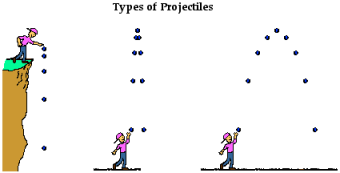KEY POINTS OF PROJECTILE MOTION:

Time of Flight, T: The time of flight depends upon two things that are angle of projection and initial velocity of an object. Moreover, the vertical displacement of an object will be zero when the point of projection and return will be on the same horizontal surface (Boundless, 2014).

Symmetry: If the point of projection and return occur along the same horizontal surface means current motion is symmetrical in the vertical plane (Boundless, 2014).

Maximum Height, H: When the vertical component of velocity, vy, will be equals to zero shows the maximum height of an object. Projectile goes against gravity as it moves up, so the velocity decelerates likewise velocity accelerates downward under gravity (Boundless, 2014).

Range of the Projectile, R: Displacement in the horizontal direction is called range. Acceleration is absent in this direction and the line of range shown when gravity only acts vertically (Boundless, 2014).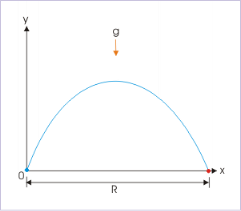As shown in this image, range of projectile is independent of the forces of gravity (Boundless, 2014).

OBJECTIVES

The purpose of this experiment was to analyze the dynamics of two dimensional projectile motion. Moreover, this was done by providing a ball with a horizontal velocity and measuring the time of flight and range. Furthermore, check the systematic errors in the data by comparing the values of vertical and horizontal acceleration.

PRIMARY OBJECTIVE: To follow the two dimensional motion of an object and to determine that the motion can be analyzed by considering the motion in each dimension separately.

SECONDARY OBJECTIVE: To check the existing possible systematic errors by comparing the horizontal acceleration with expected value of zero and vertical acceleration with the accepted value for g.

THEORY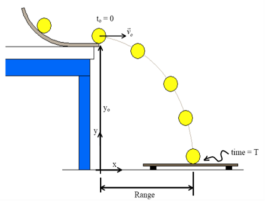Figure above is showing the experimental setup displaying measured values of initial height, range and time of flight

The ball According to the Newton’s first law of motion, there will be no acceleration in horizontal direction, unless a horizontally directed force acts on the ball. Ignoring the air friction, only force acting on the ball during flights is the force of gravity.

The range is the horizontal distance, x, between the hooter of the launcher and the place where the ball lands.

The range is given by

x = (v0 cos ø) t,

Where v0 is the initial speed of the ball as it leaves the hooter, ø is the angle of inclination above the horizontal, and t is the time of flight.

If the ball is shot horizontally (ø = 0), then the cos ø = 1 and the range is given by

x = v0 t. The time of flight will be

t = x / v0 [Equation 1]

The vertical distance, y, that the ball falls in time t is given by y = v0y t + 1/2 g t2 where v0y = 0 thus giving y = 1/2 g t2. Substituting for time, t with equation  will yield

EXPERIMENTAL METHOD

Equipment required:

1: Science Workshop Interface

2: A photo gate motion sensor

4: Projectile Launcher

5: A plastic ball

6: Measuring tape

Experimental procedure: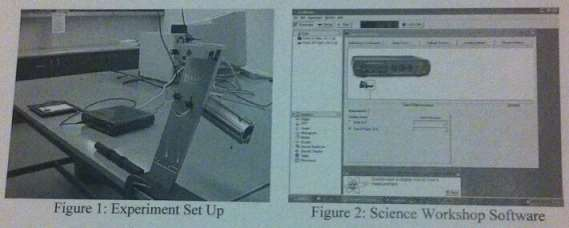Firstly, set up the angle that you have to launch by using the protractor; angles are 0, 30 or 60. Secondly, with the help of ramrod set the ball into the launcher. Moreover, the most important point is setting the right range that you wanted to calculate that is short, medium or long range. After that adjust the time of flight sensor pad, open the software “Projectile Motion” on computer and activate the application. By pulling the trigger launch the ball; note the reading for the time of flight from computer and then by using ruler measure the range. Take measurements three times for each range (short, medium, long range). Furthermore repeat the same process and take measurements three times for each angle (short range at 30 and 60).

1. How do the values for the time of flight for short, medium and long range distances compare when the ball was launched horizontally? Also compare the values with the theoretical value of time of flight at 0.
1. How do the values for the time of flight in horizontal, 30 and 60 launch compare when the ball was launched (in short range)?

DISCUSSION

Object moving in both motions that is falling free under gravity and moving both in horizontal direction is called as projectile motion; both the vertical and horizontal motions occur simultaneously but they don’t depend on each other. Moreover, after inputting the diameter of the ball into Data Studio we began our first shot at angle 0 and then according to that adjusted the landing pad to start collecting data. Furthermore, during this process we were making sure that the launcher speed remains same in every shot and also its angle; there were no problems in recording the data.

CONCLUSION

Projectile motion is two dimensional motion under constant acceleration due to gravity. Moreover, it is not necessary that an object should be thrown with some initial velocity in the horizontal direction; it is clear that there is a relationship between the angle of takeoff and the distance from which ball is thrown. Furthermore, the motion of a projectile can be studied easily by resolving it into horizontal and vertical components which are independent of each other.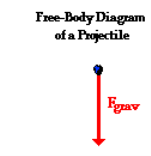STUDENT’S SUGGESTION

We have learned many things from this experiment like to calculate total time of the flight of an object and the prediction of landing point of a projectile. Moreover, Projectile motion helps in hitting the target. In addition, some of the real life examples are as follows:

A golfer needs to know at what angle above the ground the golf ball should travel to reach closer to the golf pot, there are many applications of a projectile. Real life applications of projectile include bullets on a straight spinning flight, rockets, and missiles. Furthermore, football kicked off by a player, a ball thrown by a cricketer and a missile fired from launching pad, all projected at some angles with the horizontal, are called projectiles.

References

Anonymous. (1996). the Physics Classroom. Retrieved 03 28, 2015, from physicsclassroom.com: http://www.physicsclassroom.com/class/vectors/Lesson-2/What-is-a-Projectile

Boundless. (2014, 12 12). Key Points: Range, Symmetry, Maximum Height. Retrieved 03 24, 2015, from boundless.com: https://www.boundless.com/physics/textbooks/boundless-physics-textbook/two-dimensional-kinematics-3/projectile-motion-42/key-points-range-symmetry-maximum-height-230-11284/

View all

## DMCA / Removal Request

If you are the original writer of this essay and no longer wish to have your work published on UKEssays.com then please:

Related Services

Prices from

£126

Approximate costs for:

• 1000 words
• 7 day deliveryHumanity University

Dedicated to your worth and value as a human being!

Related Lectures